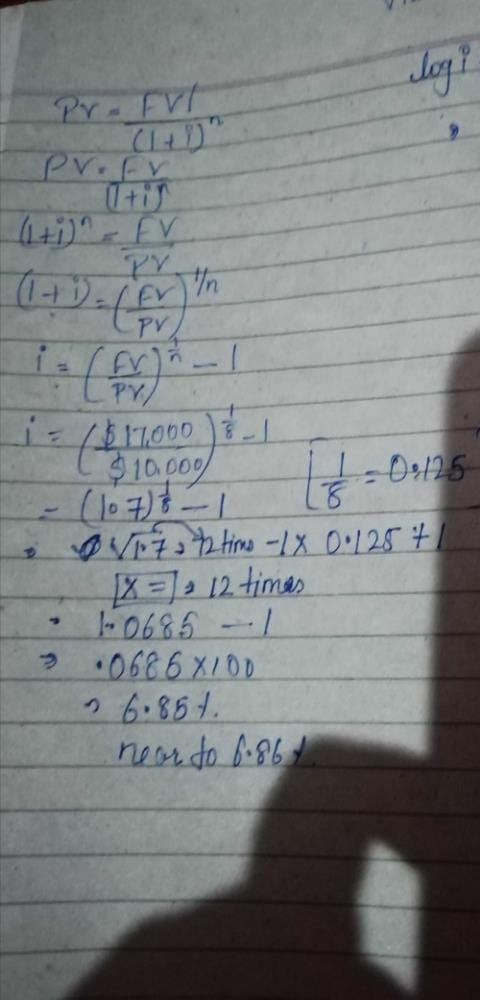Maggie deposits \$10,000 today and is promised...

### Related TestMaggie deposits \$10,000 today and is promised a return of \$17,000 in eight years. What is the implied annual rate of return?
• a)
6.86 percent
• b)
7.06 percent
• c)
5.99 percent
• d)
6.07 percentKIRAN SANGRAMEFV=PV(1+k)n
17,000=10,000(1+ k)8
8ln(1+k)=ln(1.7), therefore k=6.86%
Or using a financial calculator (TI BAII Plus),
N=8, PV= –10,000, PMT=0, FV=17,000, CPT I/Y=6.86%

 1 Crore+ students have signed up on EduRev. Have you?Priya Chaudhary
Maggie deposits \$10,000 today and is promised a return of \$17,000 in eight years. What is the implied annual rate of return?a)6.86 percentb)7.06 percentc)5.99 percentd)6.07 percentCorrect answer is option 'A'. Can you explain this answer?View courses related to this question Explore CA Foundation courses
 Explore CA Foundation coursesView courses related to this question• ### Service sector accounted for nearly ______________ percent of exports (2004... more

Question Description
Maggie deposits \$10,000 today and is promised a return of \$17,000 in eight years. What is the implied annual rate of return?a)6.86 percentb)7.06 percentc)5.99 percentd)6.07 percentCorrect answer is option 'A'. Can you explain this answer? for CA Foundation 2023 is part of CA Foundation preparation. The Question and answers have been prepared according to the CA Foundation exam syllabus. Information about Maggie deposits \$10,000 today and is promised a return of \$17,000 in eight years. What is the implied annual rate of return?a)6.86 percentb)7.06 percentc)5.99 percentd)6.07 percentCorrect answer is option 'A'. Can you explain this answer? covers all topics & solutions for CA Foundation 2023 Exam. Find important definitions, questions, meanings, examples, exercises and tests below for Maggie deposits \$10,000 today and is promised a return of \$17,000 in eight years. What is the implied annual rate of return?a)6.86 percentb)7.06 percentc)5.99 percentd)6.07 percentCorrect answer is option 'A'. Can you explain this answer?.
Solutions for Maggie deposits \$10,000 today and is promised a return of \$17,000 in eight years. What is the implied annual rate of return?a)6.86 percentb)7.06 percentc)5.99 percentd)6.07 percentCorrect answer is option 'A'. Can you explain this answer? in English & in Hindi are available as part of our courses for CA Foundation. Download more important topics, notes, lectures and mock test series for CA Foundation Exam by signing up for free.
Here you can find the meaning of Maggie deposits \$10,000 today and is promised a return of \$17,000 in eight years. What is the implied annual rate of return?a)6.86 percentb)7.06 percentc)5.99 percentd)6.07 percentCorrect answer is option 'A'. Can you explain this answer? defined & explained in the simplest way possible. Besides giving the explanation of Maggie deposits \$10,000 today and is promised a return of \$17,000 in eight years. What is the implied annual rate of return?a)6.86 percentb)7.06 percentc)5.99 percentd)6.07 percentCorrect answer is option 'A'. Can you explain this answer?, a detailed solution for Maggie deposits \$10,000 today and is promised a return of \$17,000 in eight years. What is the implied annual rate of return?a)6.86 percentb)7.06 percentc)5.99 percentd)6.07 percentCorrect answer is option 'A'. Can you explain this answer? has been provided alongside types of Maggie deposits \$10,000 today and is promised a return of \$17,000 in eight years. What is the implied annual rate of return?a)6.86 percentb)7.06 percentc)5.99 percentd)6.07 percentCorrect answer is option 'A'. Can you explain this answer? theory, EduRev gives you an ample number of questions to practice Maggie deposits \$10,000 today and is promised a return of \$17,000 in eight years. What is the implied annual rate of return?a)6.86 percentb)7.06 percentc)5.99 percentd)6.07 percentCorrect answer is option 'A'. Can you explain this answer? tests, examples and also practice CA Foundation tests.

### Related CA Foundation Content(Scan QR code)